# [機器學習] 離群值處理

2021/04/02

## 前言

Data Preprocessing(資料前處理)，是機器學習中最重要的一部分。本篇文章中可分為兩部份，前半部份算是一些對資料的觀察、分析(EDA)，後半部主要是針對特徵x進行離群值處理。

## 資料觀察

• 資料筆數： 2998
• 特徵數： 1
``````# 查看資料分布狀況
data.describe()
``````
``````count	2998.000000
mean	0.797100
std	    0.091927
min	    0.333300
25%	    0.750000
50%	    0.803750
75%	    0.864825
max	    0.960000
``````

### 箱型圖

``````plt.figure(figsize=(2,5))
plt.boxplot(data['x'],showmeans=True)
plt.title('x')
plt.show()
``````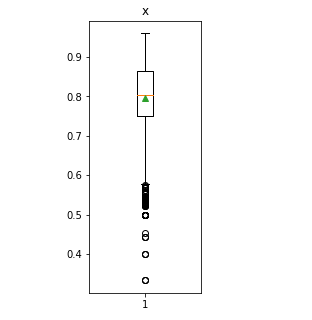### 分布狀態

``````import seaborn as sns
sns.distplot(data['x'])
plt.show()
``````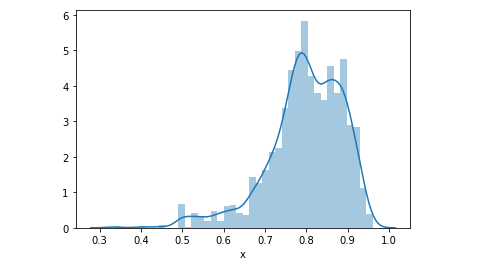### 偏態&峰度

``````#skewness and kurtosis
print("偏態(Skewness): {:.2f}".format(data['x'].skew()))
print("峰度(Kurtosis): {:.2f}".format(data['x'].kurt()))
``````
``````偏態(Skewness): -1.09

``````
• 中間圖右偏(正偏)，表示有少數幾筆資料很大，故平均 數>中位數，所以偏態係數SK>0。
• 右邊圖左偏(負偏)，表示有少數幾筆資料很小，故平均 數<中位數，所以偏態係數SK<0。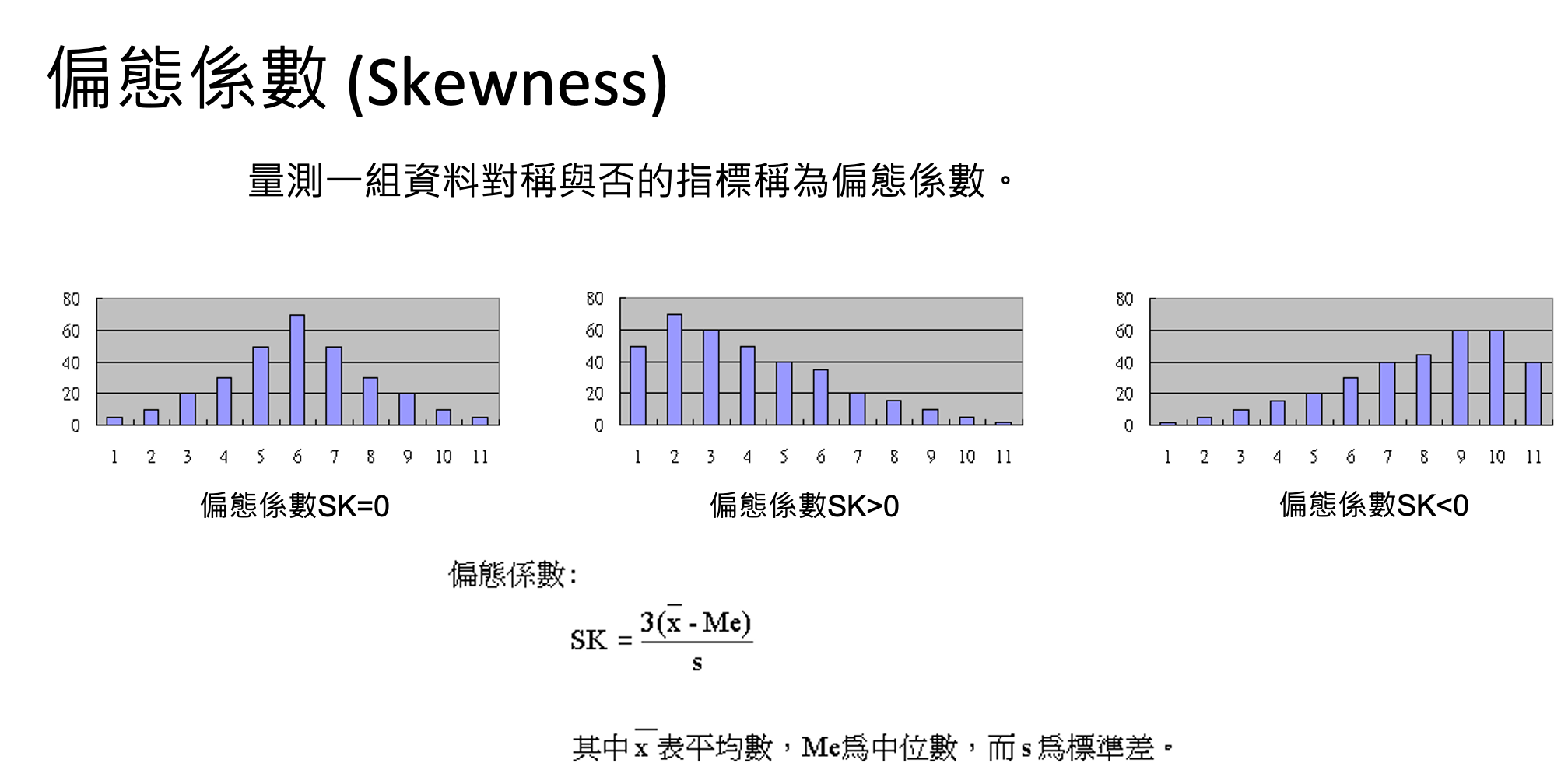## 離群值處理

### 方法1: 特徵取平方

``````exp_data = np.power(data['x'], 2)
exp_data = exp_data.replace([np.inf, -np.inf, -0], 0)
sns.distplot(exp_data)
plt.show()
``````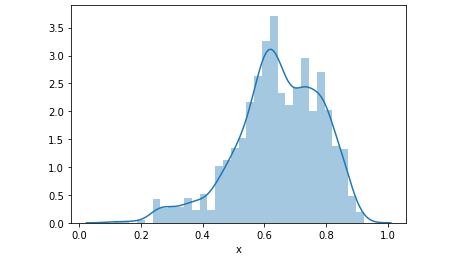### 方法二: 移除離群值``````print ("Shape Of The Before Ouliers: ",data.shape)
n=1.5
#IQR = Q3-Q1
IQR = np.percentile(data['x'],75) - np.percentile(data['x'],25)
#outlier = Q3 + n*IQR
data=data[data['x'] < np.percentile(data['x'],75)+n*IQR]
#outlier = Q1 - n*IQR
data=data[data['x'] > np.percentile(data['x'],25)-n*IQR]
print ("Shape Of The After Ouliers: ",data.shape)
``````
``````Shape Of The Before Ouliers:  (2998, 1)
Shape Of The After Ouliers:  (2897, 1)
``````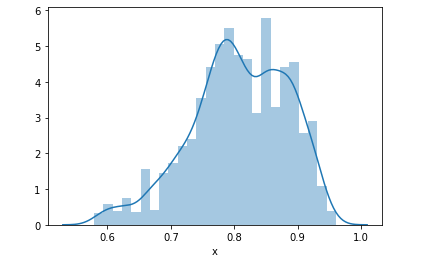https://www.youtube.com/channel/UCSNPCGvMYEV-yIXAVt3FA5A